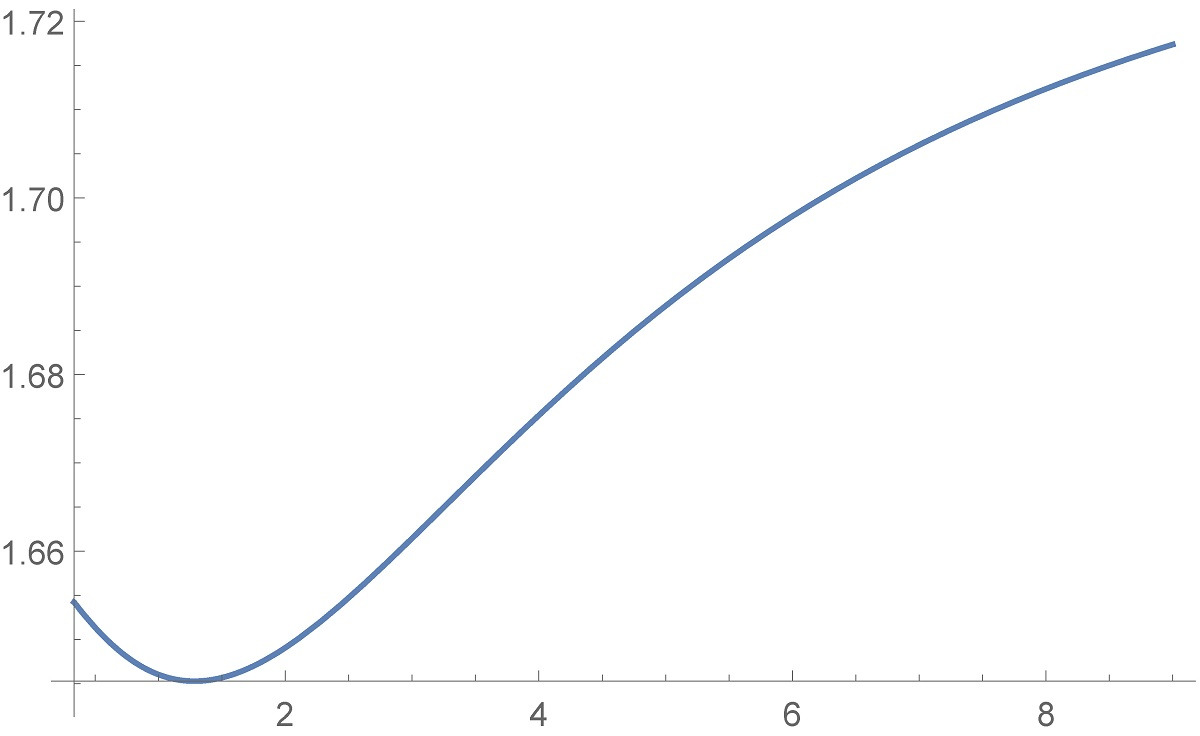Preprint Article Version 1 Preserved in Portico This version is not peer-reviewed

# Complete monotonicity of a difference constituted by four derivatives of a function involving trigamma function

Version 1 : Received: 10 November 2020 / Approved: 12 November 2020 / Online: 12 November 2020 (11:58:39 CET)

How to cite: Qi, F. Complete monotonicity of a difference constituted by four derivatives of a function involving trigamma function. Preprints 2020, 2020110343 (doi: 10.20944/preprints202011.0343.v1). Qi, F. Complete monotonicity of a difference constituted by four derivatives of a function involving trigamma function. Preprints 2020, 2020110343 (doi: 10.20944/preprints202011.0343.v1).

## Abstract

In the paper, by virtue of convolution theorem for the Laplace transforms, Bernstein's theorem for completely monotonic functions, and other techniques, the author finds necessary and sufficient conditions for a difference constituted by four derivatives of a function involving trigamma function to be completely monotonic.## Subject Areas

complete monotonicity; necessary and sufficient condition; difference; derivative; trigamma function; convolution theorem for the Laplace transforms; Bernstein's theorem for completely monotonic functions

Views 0